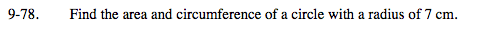### Home > MC2 > Chapter 9 > Lesson 9.3.1 > Problem9-78

9-78.

Find the area and circumference of a circle with a radius of 7 cm. Homework Help ✎Area of a circle = r2 π
Circumference = 2πr

Substitute 7 for r in each equation.

The area is approximately 159.9 cm2.
What is the perimeter?